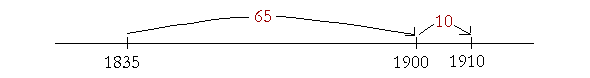S k i l l
i n
A R I T H M E T I C

Lesson 7  Section 3

# THE MEANING OF SUBTRACTION

## Mental calculation:Completing 100  and  Rounding off

Back to Section 1

Problem.   If an item costs \$.79 and you give a dollar bill, how much is your change?

.79 + .21 = 1.00

("79 + 20 = 99; plus 1 is 100.")

In other words:

 6. How do we complete 100? 64 + ? = 100 Add tens to get into the 90's, then add ones to complete 100.

Example 1.   64 + ? = 100

Solution.   We must get into the 90's.  64 + 30 brings us to 94.  Plus 6 is 100.

64 + 36 = 100

36 is called the complement to 100 of 64 because 36 completes 64 to make 100.

Notice that the tens digits, 6 + 3, add up to 9 tens -- they bring us to the 90's.  While the ones digits, 4 + 6, add up to 10.

Example 2.   In a recent election, 58% voted for Smith.  What percent did not vote for Smith?

Answer.  100% is the whole. (Lesson 3, Question 5.)  So the question is,

58 + ? = 100

58 + 40 brings us to 98.   Plus 2 is 100.

42% did not vote for Smith.

Again, the tens digits, 5 + 4, add up to 9 tens -- that brings us to the 90's; while the ones digits, 8 + 2, add up to 10.

Example 3.   How much is 104 − 86?

Technique.  First, complete 100:86 + 14 will bring us to 100.  Plus 4 is 104.

Thus the distance between 86 and 104 is

14 + 4 = 18.

Example 4.   Mark Twain was born in 1835 and died in 1910.  How old was he when he died?

Answer.  Jump first to the century mark.  Say,

"1835 plus 65 is 1900, plus 10 is 1910.  He was 75."   (65 + 10)Example 5.   How many years passed between the discovery of America in 1492 and the signing of the Declaration of Independence in 1776?

Solution.  What must we add to 1492 to get 1776?1492 + 200 brings us to 1692.  Plus 8 is 1700.  Plus 76 is 1776.

208 + 76 = 284 years

Rounding off

What is an easy way to subtract 95 from 261?

261 − 95 = ?

Rather than subtract 95, subtract 100.  To do that, we must add 5 to 95.  Therefore to preserve the difference -- which is the distance between the two numbers -- we must also add 5 to 261.

261 − 95 = 266 − 100 = 166.The distance from 95 to 261 is equal to the distance from 100 to 266.

In subtraction, if we add the same number to both terms,
the difference -- the distance between them -- will not change.

 7. How do we subtract by rounding off? 96 − 18 = 98 − 20 \$18.00 − \$2.95 = \$18.05 − \$3.00 Add to the smaller number to make it a multiple of 10; or, if it is a decimal or a fraction, make it a whole number. To preserve the difference, add that same number to the larger. Or, subtract and then add that number to the answer.

Example 6.    674 − 19

Add 1 to both 19 and 674.  Say,

Example 6.   "675 minus 20 is 655."

Or, say

Example 6.   "674 minus 20 is 654. Plus 1 is 655."

Example 7.    85 − 38

Example 7.   "87 − 40 = 47."

Or, if we add only to 38, then

Example 7.   "85 − 40 = 45,  plus 2 is 47."

Example 8.  The cents in the smaller number are less.

8.733.29

29 cents is less than 73 cents.  In this case, subtract the cents first.  Round off to 30 cents and say,

"74 minus 30 is 44.   5.44."

(3  from  8  is  5.)

Example 9.  The cents in the smaller number are more.

\$48.00 − \$15.90

90 cents are more than no cents. In this case, round off to the next whole dollar. Say,

"\$48 − \$16 is \$32,

plus 10 cents is \$32.10."

Example 10.   9.102.79

Round off to the next whole dollar -- by adding the complement to 100 of the 79 cents.

"9.10 minus 3.00 is 6.10,

plus 21 cents is 6.31."

21¢ is the complement to 100 of 79¢.

Example 11.   How much is \$115 less 10%?

Solution.   10% of \$115 is \$11.50. (Lesson 4.)  Therefore say,

"\$115 minus \$12 is \$103;

plus 50 cents is \$103.50."

Example 12.   You have \$374.12 in your checking account, and you write a check for \$48.70.  What is your new balance?

Answer.  Speak"\$374.12 minus 50 dollars is \$324.12,

plus \$1.30 is \$325.42."

Faster than a calculatorPlease "turn" the page and do some Problems.

or

Continue on to the next Lesson.

Section 1 of this Lesson

Section 2